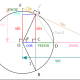# How Dirac Made Every Number

A couple weeks back I offered a challenge taken from Graham Farmelo’s biography (The Strangest Man) of the physicist Paul Dirac. The physicist had been invited into a game to create whole numbers by using exactly four 2’s and the normal arithmetic operations, for example:$1 = \frac{2 + 2}{2 + 2}$$2 = 2^{2 - \left(2 \div 2\right)}$$4 = 2^2 \div 2 + 2$$8 = 2^{2^{2}} \div 2$

While four 2’s have to be used, and not any other numerals, it’s permitted to use the 2’s stupidly, as every one of my examples here does. Dirac went off and worked out a scheme for producing any positive integer from them. Now, if all goes well, Dirac’s answer should be behind this cut and it hasn’t been spoiled in the reader or the mails sent out to people reading it.

Dirac’s formula, according to Farmelo, is this:$n = -\log_{2}\left( \log_{2}\left( \sqrt{ \sqrt{ \cdots \sqrt{2}} } \right) \right)$

You should have n square roots in that innermost logarithm; that is, for example,$1 = -\log_{2}\left( \log_{2}\left( \sqrt{2} \right) \right)$$2 = -\log_{2}\left( \log_{2}\left( \sqrt{\sqrt{2}} \right) \right)$$4 = -\log_{2}\left( \log_{2}\left( \sqrt{\sqrt{\sqrt{\sqrt{2}}}} \right) \right)$$8 = -\log_{2}\left( \log_{2}\left( \sqrt{\sqrt{\sqrt{\sqrt{\sqrt{\sqrt{\sqrt{\sqrt{2}}}}}}}} \right) \right)$

This is a magnificent solution, obviously, and it’s a good problem for your pre-algebra class to show why it works, at least for some particular number. It does make a slightly stupid use of one of the 2’s, since there’s no discernable difference between$\sqrt{ }$ and$\sqrt{ }$, but that’s within the rules.

The system can be extended to other whole powers of two, so if you want to build any integer using exactly four 4’s, or 16’s, or 1024’s, you’re in luck, but I admit I don’t see how this gets you to building any number out of a string of four 3’s. The square root symbol can be traced back to the early 16th century (and a forerunner, RR, to Leonardo da Pisa in about 1220), and extensions of it to take cube or fifth or other roots have always been available, but by writing the number of the root somewhere around the base symbol. Dirac’s scheme works for 2 (and powers of 2) because a square root symbol by itself carries that 2 implicitly; cube roots require the 3 be written explicitly.## Author: Joseph Nebus

I was born 198 years to the day after Johnny Appleseed. The differences between us do not end there. He/him.

## 10 thoughts on “How Dirac Made Every Number”

1.delarsea says:

Reblogged this on How and Why?.

Like

2.abyssbrain says:

Nice post. I’m not familiar with the history of this problem and that it’s attributed the Dirac. Though I have seen the version of using the 4’s to produce every number.

Like

1.Joseph Nebus says:

I don’t know that the problem traces to Dirac, but at least a biography of him did credit him with a solution using exactly four 2’s. Using 4’s to make numbers is another wonderful puzzle, though.

Liked by 1 person

This site uses Akismet to reduce spam. Learn how your comment data is processed.